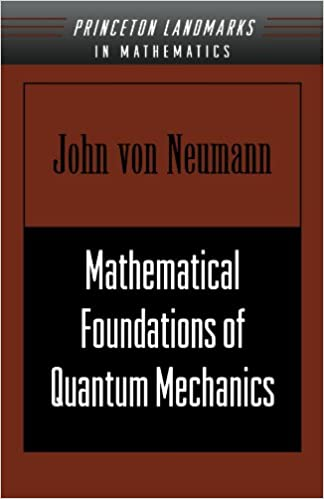# Mathematical Foundations of Quantum MechanicsEnglish | ISBN: 0691028931 464 pages | PDF | 88 MB

Mathematical Foundations of Quantum Mechanics was a revolutionary book that caused a sea change in theoretical physics. Here, John von Neumann, one of the leading mathematicians of the twentieth century, shows that great insights in quantum physics can be obtained by exploring the mathematical structure of quantum mechanics. He begins by presenting the theory of Hermitean operators and Hilbert spaces. These provide the framework for transformation theory, which von Neumann regards as the definitive form of quantum mechanics. Using this theory, he attacks with mathematical rigor some of the general problems of quantum theory, such as quantum statistical mechanics as well as measurement processes. Regarded as a tour de force at the time of publication, this book is still indispensable for those interested in the fundamental issues of quantum mechanics.